# Concurrent vs Sequential Combinational vs Sequential logic Combinational

• Slides: 21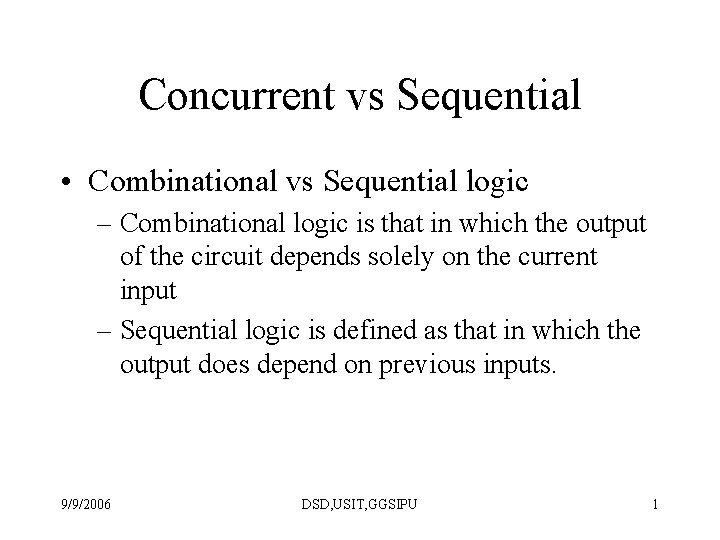Concurrent vs Sequential • Combinational vs Sequential logic – Combinational logic is that in which the output of the circuit depends solely on the current input – Sequential logic is defined as that in which the output does depend on previous inputs. 9/9/2006 DSD, USIT, GGSIPU 1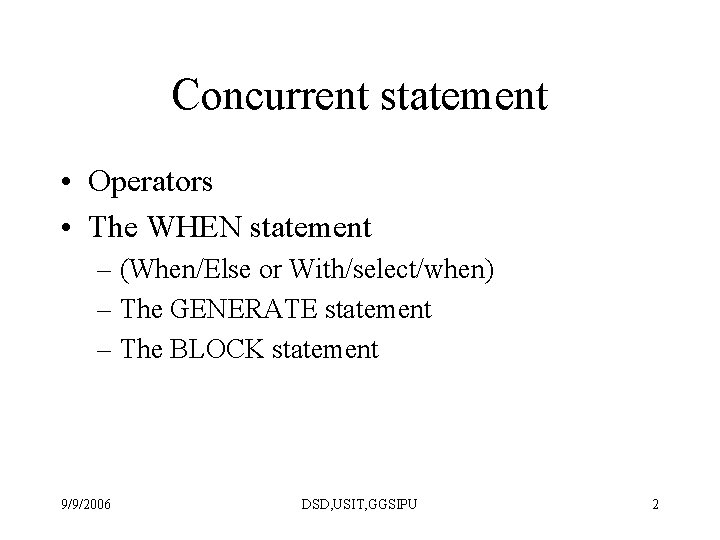Concurrent statement • Operators • The WHEN statement – (When/Else or With/select/when) – The GENERATE statement – The BLOCK statement 9/9/2006 DSD, USIT, GGSIPU 2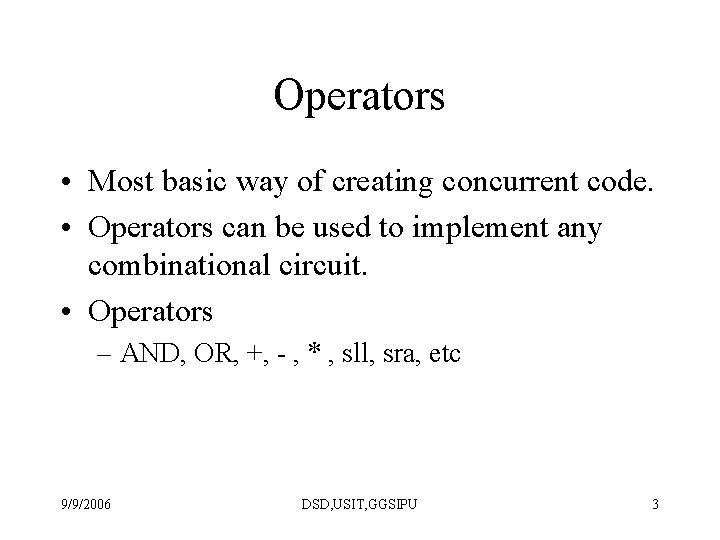Operators • Most basic way of creating concurrent code. • Operators can be used to implement any combinational circuit. • Operators – AND, OR, +, - , * , sll, sra, etc 9/9/2006 DSD, USIT, GGSIPU 3Operators Type Data types Logical NOT, AND, NOR, XOR, XNOR Bit, Bit_vector Std_logic, std_logic_vector Std_ulogic_vector, std_ulogic Arithmetic +, -, *, /, ** Integer, signed, unsigned Comparison =, /=, <, >, <=, >= All above Shift Sll, srl, sla, sra, rol, ror Bit_vector Concatenation &, (, , , ) Same as above 9/9/2006 DSD, USIT, GGSIPU 4When (Simple and selected) • Fundamental concurrent statement • Syntax assignment WHEN condition ELSE ………. ; 9/9/2006 DSD, USIT, GGSIPU 5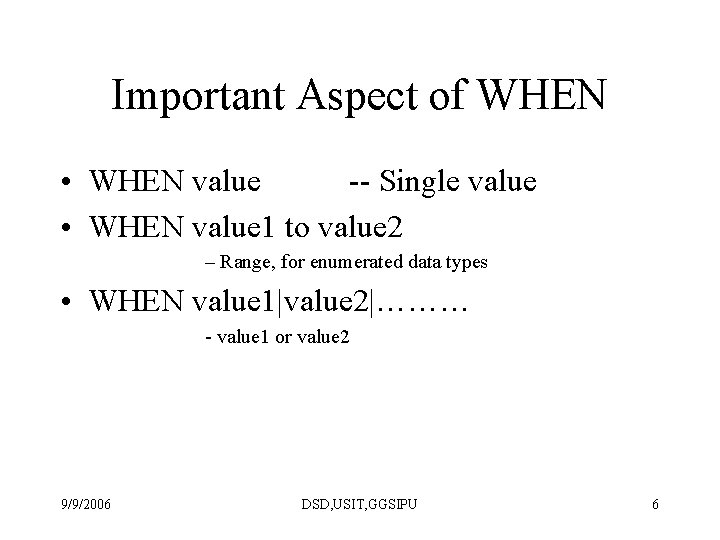Important Aspect of WHEN • WHEN value -- Single value • WHEN value 1 to value 2 – Range, for enumerated data types • WHEN value 1|value 2|……… - value 1 or value 2 9/9/2006 DSD, USIT, GGSIPU 6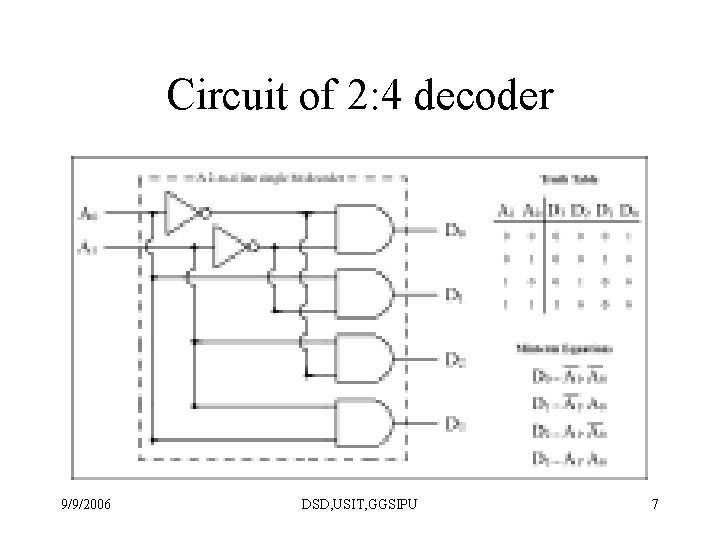Circuit of 2: 4 decoder 9/9/2006 DSD, USIT, GGSIPU 73: 8 Decoder • • • entity decodedr 38 is Port ( a : in std_logic_vector(2 downto 0); b : out std_logic_vector(7 downto 0); en : in std_logic); end decodedr 38; • • • architecture Behavioral of decodedr 38 is signal temp : std_logic_vector(7 downto 0); begin temp <= "00000001" when a="000" else "00000010" when a="001" else "00000100" when a="010" else "00001000" when a="011" else "00010000" when a="100" else "00100000" when a="101" else "01000000" when a="110" else "10000000"; • • • b <= temp when en='1' else (others => '0'); end Behavioral; 9/9/2006 DSD, USIT, GGSIPU 8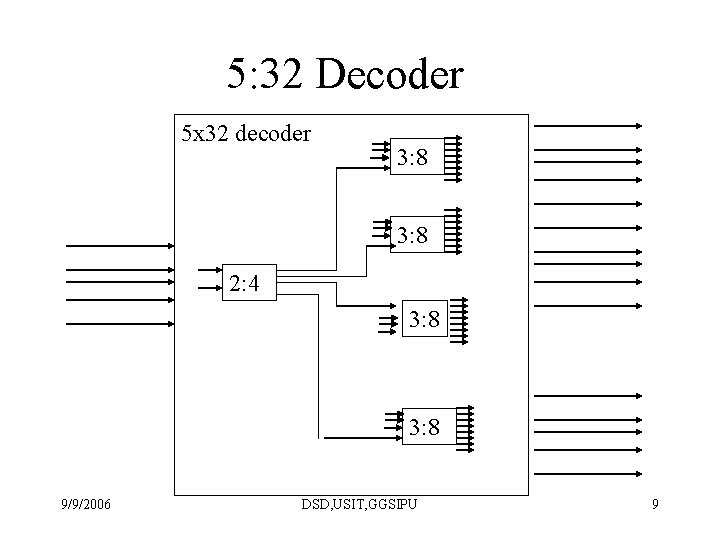5: 32 Decoder 5 x 32 decoder 3: 8 2: 4 3: 8 9/9/2006 DSD, USIT, GGSIPU 9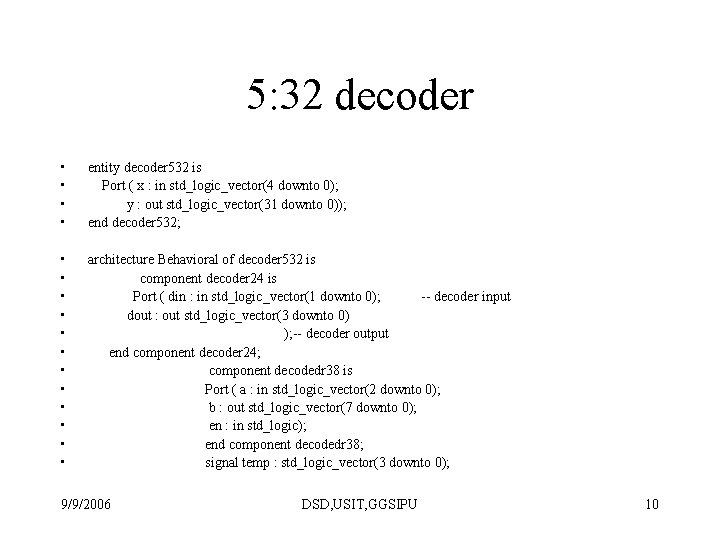5: 32 decoder • • entity decoder 532 is Port ( x : in std_logic_vector(4 downto 0); y : out std_logic_vector(31 downto 0)); end decoder 532; • • • architecture Behavioral of decoder 532 is component decoder 24 is Port ( din : in std_logic_vector(1 downto 0); -- decoder input dout : out std_logic_vector(3 downto 0) ); -- decoder output end component decoder 24; component decodedr 38 is Port ( a : in std_logic_vector(2 downto 0); b : out std_logic_vector(7 downto 0); en : in std_logic); end component decodedr 38; signal temp : std_logic_vector(3 downto 0); 9/9/2006 DSD, USIT, GGSIPU 105: 32 decoder (cont. . ) • • begin u 1: decoder 24 port map(din(1)=>x(4), din(0)=>x(3), dout(3)=>temp(3), dout(2)=>temp(2), dout(1)=>temp(1), dout(0)=>te mp(0)); u 2: decodedr 38 port map(en=>temp(0), a(2)=>x(2), a(1)=>x(1), a(0)=>x(0), b(7)=>y(7), b(6)=>y(6), b(5)=>y(5), b(4)=>y(4), b(3)=>y(3), b(2)=>y(2), b(1)=>y(1), b(0)=>y(0)); u 3: decodedr 38 port map(en=>temp(1), a(2)=>x(2), a(1)=>x(1), a(0)=>x(0), b(7)=>y(15), b(6)=>y(14), b(5)=>y(13), b(4)=>y( 12), b(3)=>y(11), b(2)=>y(10), b(1)=>y(9), b(0)=>y(8)); u 4: decodedr 38 port map(en=>temp(2), a(2)=>x(2), a(1)=>x(1), a(0)=>x(0), b(7)=>y(23), b(6)=>y(22), b(5)=>y(21), b(4)=>y( 20), b(3)=>y(19), b(2)=>y(18), b(1)=>y(17), b(0)=>y(16)); u 5: decodedr 38 port map(en=>temp(3), a(2)=>x(2), a(1)=>x(1), a(0)=>x(0), b(7)=>y(31), b(6)=>y(30), b(5)=>y(29), b(4)=>y( 28), b(3)=>y(27), b(2)=>y(26), b(1)=>y(25), b(0)=>y(24)); end Behavioral; 9/9/2006 DSD, USIT, GGSIPU 11Conditional Signal Assignment statement • The conditional signal assignment statement selects different values for the target signal based on the specified, possibly different, conditions. A typical syntax – target-signal <= [waveform-elements when condition else. . Waveform-elements when condition]; 9/9/2006 DSD, USIT, GGSIPU 12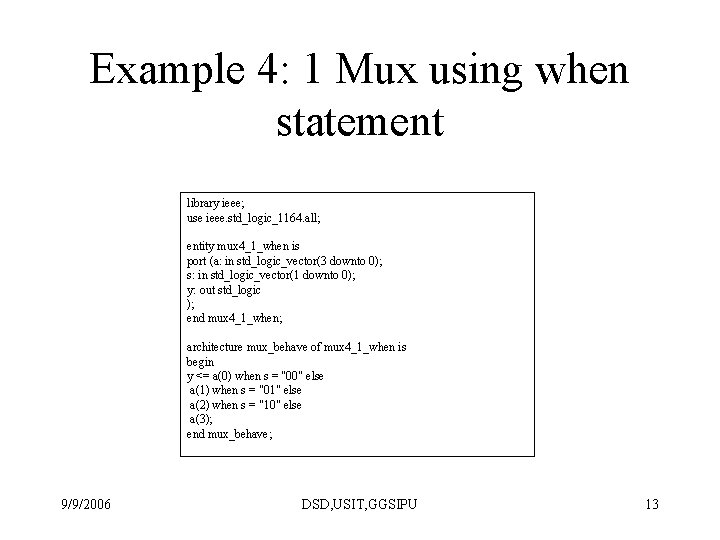Example 4: 1 Mux using when statement library ieee; use ieee. std_logic_1164. all; entity mux 4_1_when is port (a: in std_logic_vector(3 downto 0); s: in std_logic_vector(1 downto 0); y: out std_logic ); end mux 4_1_when; architecture mux_behave of mux 4_1_when is begin y <= a(0) when s = "00" else a(1) when s = "01" else a(2) when s = "10" else a(3); end mux_behave; 9/9/2006 DSD, USIT, GGSIPU 13Symbol of 2: 1 Mux 9/9/2006 DSD, USIT, GGSIPU 14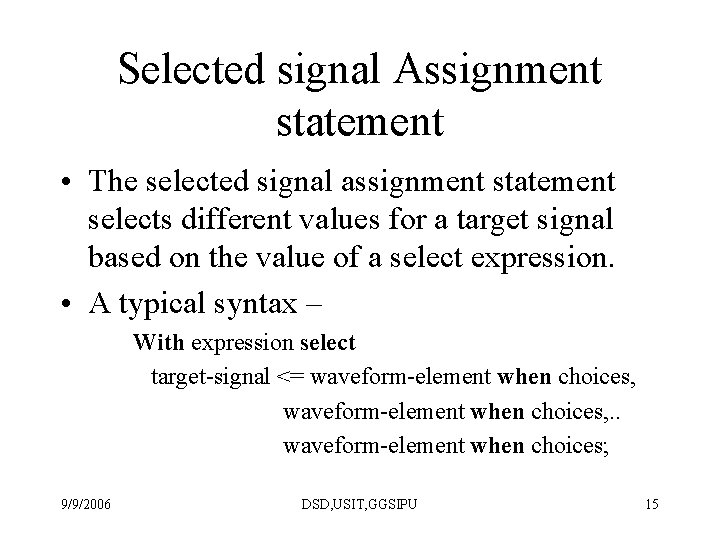Selected signal Assignment statement • The selected signal assignment statement selects different values for a target signal based on the value of a select expression. • A typical syntax – With expression select target-signal <= waveform-element when choices, . . waveform-element when choices; 9/9/2006 DSD, USIT, GGSIPU 15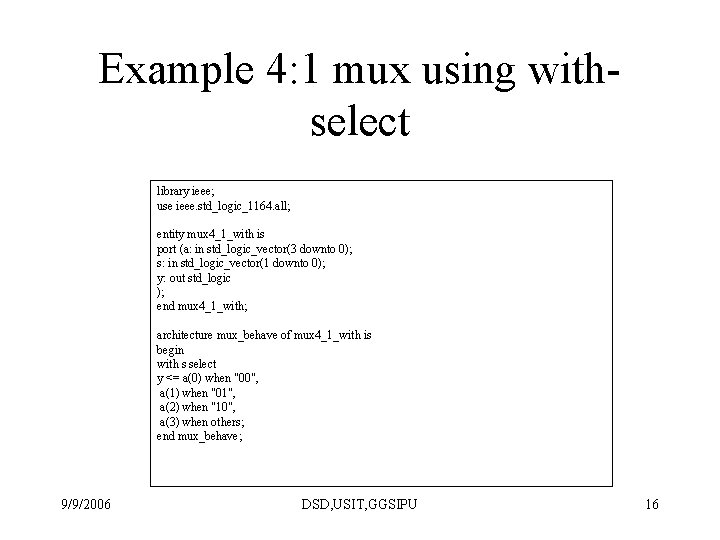Example 4: 1 mux using withselect library ieee; use ieee. std_logic_1164. all; entity mux 4_1_with is port (a: in std_logic_vector(3 downto 0); s: in std_logic_vector(1 downto 0); y: out std_logic ); end mux 4_1_with; architecture mux_behave of mux 4_1_with is begin with s select y <= a(0) when "00", a(1) when "01", a(2) when "10", a(3) when others; end mux_behave; 9/9/2006 DSD, USIT, GGSIPU 16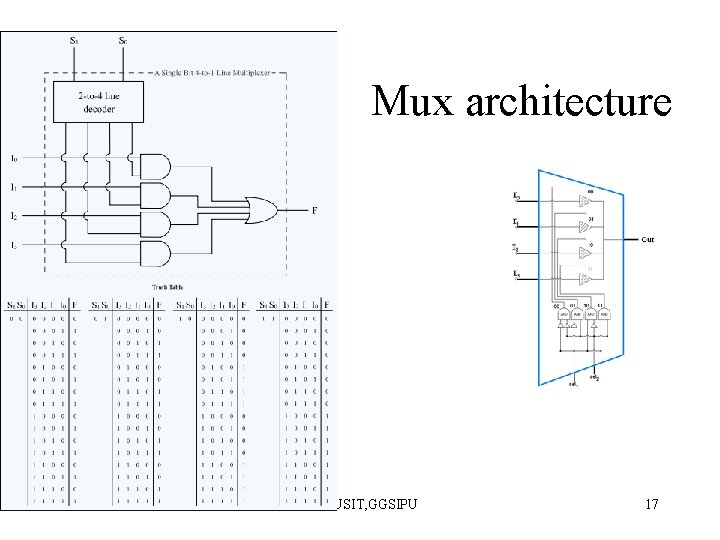Mux architecture 9/9/2006 DSD, USIT, GGSIPU 17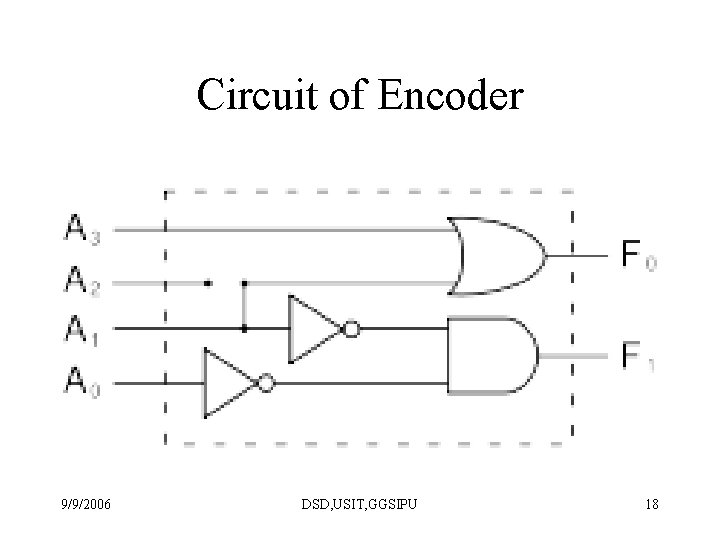Circuit of Encoder 9/9/2006 DSD, USIT, GGSIPU 18Truth table of encoder 9/9/2006 A 3` 0 A 2 0 A 1 0 A 0 1 F 1 0 F 0 0 1 0 1 0 0 0 1 1 DSD, USIT, GGSIPU 19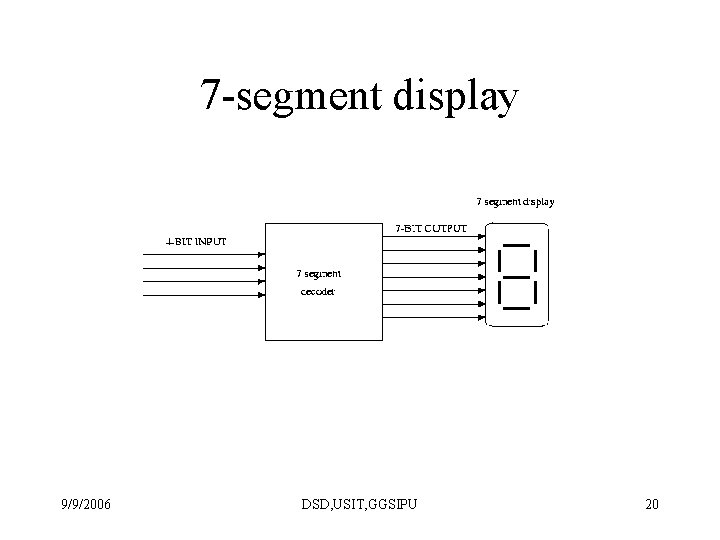7 -segment display 9/9/2006 DSD, USIT, GGSIPU 20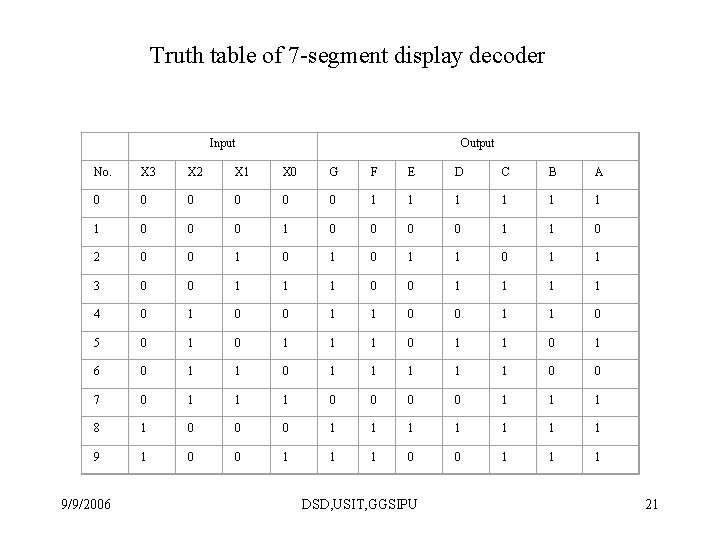Truth table of 7 -segment display decoder Input Output No. X 3 X 2 X 1 X 0 G F E D C B A 0 0 0 1 1 1 1 0 0 0 0 1 1 0 2 0 0 1 0 1 1 3 0 0 1 1 1 1 4 0 1 0 0 1 1 0 5 0 1 1 1 0 1 6 0 1 1 1 1 1 0 0 7 0 1 1 1 0 0 1 1 1 8 1 0 0 0 1 1 1 1 9 1 0 0 1 1 1 9/9/2006 DSD, USIT, GGSIPU 21